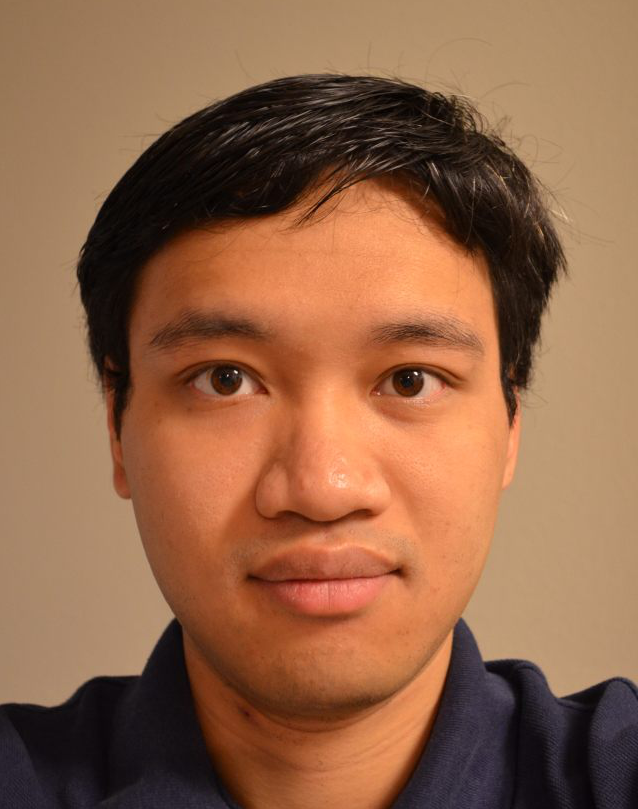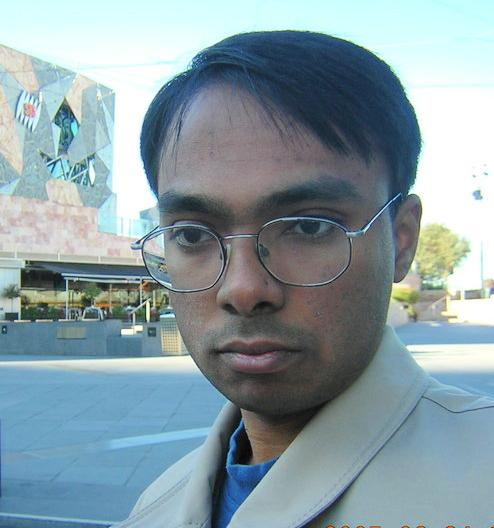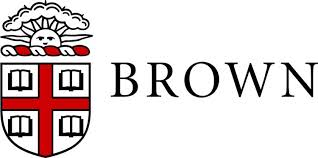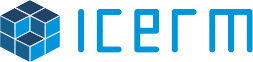Information

The first Sublinear Algorithms and Big Data Day or, in short, "The Sublinear Day" will take place at Brown ICERM on Friday, April 18. Further details are below.

This event will bring together researchers from academic institutions in New England for a day of interaction and discussion. The Sublinear Day will feature four hour-long + two 30-minute presentations by leading experts in the areas of sublinear and streaming algorithms.

Schedule

• 09:00 – 09:30

Coffee Break

• 09:30 – 10:15
Ronitt Rubinfeld (Massachusetts Institute of Technology)
Local computation algorithms for sparse graphs

Consider a setting in which inputs to and outputs from a computational problem are so large, that there is not time to read them in their entirety. However, if one is only interested in small parts of the output at any given time, is it really necessary to solve the entire computational problem? Is it even necessary to view the whole input? We describe recent work in the model of {\em local computation algorithms} which for a given input, supports queries by a user to values of specified bits of a legal output. The goal is to design local computation algorithms in such a way that very little of the input needs to be seen in order to determine the value of any single bit of the output. In this talk, we describe a number of graph problems for which sublinear time and space local computation algorithms have been developed.

This talk will describe joint work with several researchers, including Noga Alon, Gil Tamir, Shai Vardi, Ning Xie, Reut Levi, Dana Ron, Andrea Campagna, and Alan Guo.

• 10:30 – 10:55
Michael Kapralov (Massachusetts Institute of Technology)
Approximating matching size from random streams

We present a streaming algorithm that makes one pass over the edges of an unweighted graph presented in random order, and produces a polylogarithmic approximation to the size of the maximum matching in the graph, while using only polylogarithmic space. Prior to this work the only approximations known were a folklore $$\tilde{O}(\sqrt{n})$$-approximation with polylogarithmic space in an $$n$$-vertex graph and a constant approximation with $$\Omega(n)$$ space. Our work thus gives the first algorithm where both the space and approximation factors are smaller than any polynomial in $$n$$.

This is joint work with Sanjeev Khanna and Madhu Sudan.

• 11:00 – 11:25
Huy Le Nguyen (Princeton University)
Turnstile Streaming Algorithms Might as Well Be Linear Sketches

In the turnstile model of data streams, an underlying vector x in $$\{-m, -m + 1, ..., m - 1, m\}^n$$ is presented as a long sequence of arbitrary positive and negative integer updates to its coordinates. A randomized algorithm seeks to approximate a function $$f(x)$$ with constant probability while only making a single pass over this sequence of updates and using a small amount of space. All known algorithms in this model are linear sketches: they sample a matrix $$A$$ from a distribution on integer matrices in the preprocessing phase, and maintain the linear sketch $$Ax$$ while processing the stream. At the end of the stream, they output an arbitrary function of $$Ax$$. One cannot help but ask: are linear sketches universal?

In this work we answer this question by showing that any 1-pass constant probability streaming algorithm for approximating an arbitrary function $$f$$ of $$x$$ in the turnstile model can also be implemented by sampling a matrix $$A$$ from the uniform distribution on $$O(n log m)$$ integer matrices, with entries of magnitude $$poly(n)$$, and maintaining the linear sketch $$Ax$$. Furthermore, the logarithm of the number of possible states of $$Ax$$, as $$x$$ ranges over $$\{-m, -m + 1, ...,m\}^n$$, plus the amount of randomness needed to store $$A$$, is at most a logarithmic factor larger than the space required of the space-optimal algorithm. Our result shows that to prove space lower bounds for 1-pass streaming algorithms, it suffices to prove lower bounds in the simultaneous model of communication complexity, rather than the stronger 1-way model. Moreover, the fact that we can assume we have a linear sketch with polynomially-bounded entries further simplifies existing lower bounds, e.g., for frequency moments we present a simpler proof of the $$\tilde{\Omega}(n^{1-2/k})$$ bit complexity lower bound without using communication complexity.

Joint work with Yi Li and David P. Woodruff.

•  11:30 – 13:00

Lunch Break

• 13:00 – 13:45
Sofya Raskhodnikova (Pennsylvania State University + Boston University)
Testing and Reconstruction of Lipschitz Functions

We will study algorithmic questions about a basic property of functions: the Lipschitz property. The Lipschitz property is defined as follows: a real-valued function $$f$$ that takes a vector $$x$$ is Lipschitz if for all pairs of inputs $$x$$ and $$y$$, the value $$|f(x)-f(y)|$$ is at most the number of coordinates on which $$x$$ and $$y$$ differ. We will discuss sublinear-time algorithms for testing whether a function is Lipschitz and for reconstructing Lipschitz functions.

We will show applications of these algorithms to differential privacy. The framework of differential privacy allows one to answer global queries about information in a database while protecting privacy specific to individuals whose information it contains. There are many known differentially private algorithms for specific queries and classes of queries. What if database analysts are allowed to write their own programs to access a private database? Can one test if such programs are differentially private? Can one ensure that they are differentially private? We will show that sublinear-algorithms for testing and reconstructing Lipschitz functions can be used to answer these question.

The talk is based on joint works with Madhav Jha, with Pranjal Awasthi, Madhav Jha and Marco Molinaro, and with Kashyap Dixit, Madhav Jha and Abhradeep Thakurta.

• 14:00 – 14:45
Andrew McGregor (University of Massachusetts, Amherst)
Analyzing Big Graphs via Sketching and Streaming

Early work on data stream algorithms focused on estimating numerical statistics such as quantiles, frequency moments, and heavy hitters given limited memory and a single pass over the input. However, there's now a growing body of work on analyzing more structured data such as graphs. In this talk, we survey recent research in this area.

• 15:00 – 15:45
Amit Chakrabarti (Dartmouth College)
Streaming Interactive Proofs or: How I Learned to Stop Worrying and Trust the Cloud

We consider the following scenario motivated by the rise of cloud computing. A client needs to process a massive stream of data, arriving online, but is unable to store even a small fraction of the data. It outsources the processing to a commercial cloud computing service, but is unwilling to blindly trust answers returned by this service. How can we design a suitable communication protocol between client and server that allows the server to not just provide the desired answer but prove to the client that the answer is correct?

This general question has been the subject of several recent research works. In this talk we shall focus on one particular problem of great practical interest: Nearest Neighbour Search. Our main protocol allows a client to find, with proof (in the above sense) the nearest neighbour to a query point in a stream of data points, while storing barely more than a constant number of points. In fact, the protocol we design is considerably more general, allowing us to solve many other data streaming problems, and leading to a rich theory within communication complexity, known as Arthur-Merlin communication. The talk will outline this theory broadly.

Based on joint work with Cormode, McGregor, Thaler, and Venkatasubramanian.

• 15:45 – 16:00
Coffee Break

Speakers

•Ronitt Rubinfeld

Ronitt Rubinfeld received her PhD at the University of California, Berkeley, and is currently on the faculties at MIT and Tel Aviv University. Her research focuses on sub-linear time algorithms for big data sets.

•Michael Kapralov

Michael Kapralov is a postdoc in the Theory of Computation Group at MIT. His research interests are in classical combinatorial optimization problems as well as problems motivated by modern data models, such as streaming, sketching and online algorithms. More recent interests include sparse recovery and Fourier sampling. Michael obtained his Ph.D. from Stanford University in 2012.

•Huy Le Nguyen

Huy Le Nguyen is a graduate student in the Computer Science Department at Princeton University, advised by Professor Moses Charikar. Before attending Princeton, he received his Bachelor's degrees in Computer Science and Mathematics, and Master of Engineering in Computer Science, all from MIT. His research has focused on algorithms for large datasets, including dimensionality reduction methods, approximate nearest neighbor search, and fast numerical linear algebra algorithms. He is also interested in other areas of theoretical computer science, and has worked on online algorithms, natural algorithms, and data structure lower bounds.

•Sofya Raskhodnikova is an associate professor of Computer Science and Engineering at the Pennsylvania State University. She is currently on sabbatical, visiting Boston University and Harvard University. Sofya works on design and analysis of sublinear-time algorithms for combinatorial problems and on privacy-preserving methods for publishing aggregate statistical data. Sofya got her PhD from MIT in 2003. From the fall of 2003 to 2006, she worked at the Hebrew University of Jerusalem, the Weizmann Institute of Science and the Institute for Pure and Applied Mathematics at UCLA.

•Andrew McGregor

Andrew McGregor is an Assistant Professor at the University of Massachusetts, Amherst. He received a B.A. degree and the Certificate of Advance Study in Mathematics from the University of Cambridge and a Ph.D. from the University of Pennsylvania. He also spent a couple of years as a post-doc at UC San Diego and Microsoft Research SVC. He is interested in many areas of theoretical computer science and specializes in data stream algorithms, linear sketching, and communication complexity. He received the NSF Career Award in 2010.

•Amit Chakrabarti

Amit Chakrabarti is an Associate Professor in the Department of Computer Science at Dartmouth College. He received an M.A. and a Ph.D. in Computer Science from Princeton University in 2002 and a B.Tech. in Computer Science from the Indian Institute of Technology, Bombay, along with the President of India Gold Medal, in 1997. Professor Chakrabarti's research is in the broad area of theoretical computer science. Specific interests are (1) complexity theory, especially communication complexity and the application of information theory, and (2) algorithms, including space-efficient algorithms for massive data streams and approximation techniques for optimization problems.

Organizers and Support

Organizers:

Program + Homepage: Grigory Yaroslavtsev
Local arrangements: Lauren Warmington
Coordinator: Nicole Henrichs
Technical support: Isani Cayetano

Supported by: